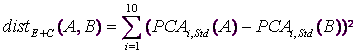﻿ 5. How To use « TIMi – StarDust module »? > 5.5. How to create a segmentation? > 5.5.1. Definition of the Distance to use inside the segmentation

# 5.5.1. Definition of the Distance to use inside the segmentation

A segmentation is based on a “distance-definition”.

Using StarDust, you can create very complex distance-definition. For example, the following axis can be included inside you distance-definition:

On all continuous variables:

oNon-normalized original axis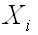of the data: select “ED:none” inside the “Normalize” column inside the large table on the “Standardization” tab inside the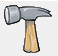parameter window (this tab is NOT accessible with the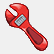button, only with thebutton. See illustration: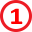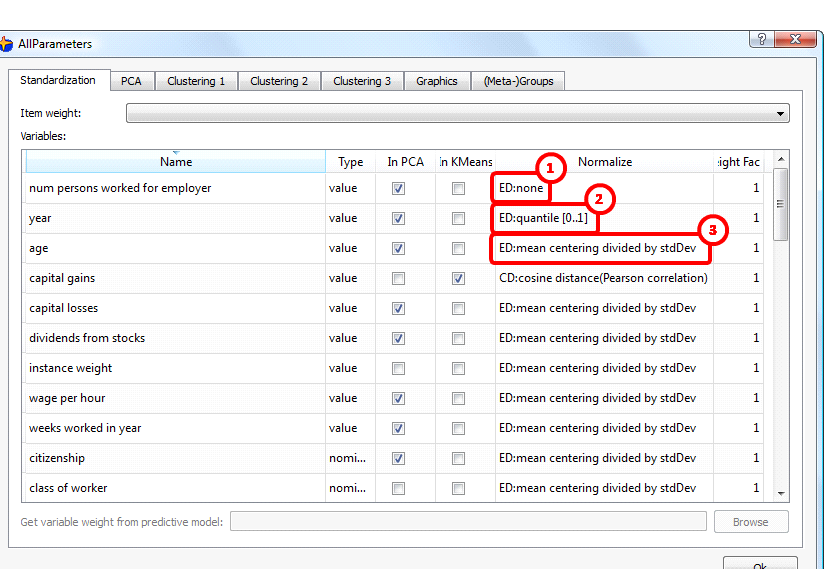oNormalized-axis. There are two type of normalization:

Standard Normalization for inclusion inside an Euclidean-Distance: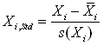... where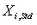is the ith column of the dataset that has been normalized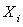is the ith column of the dataset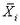is the mean of the ith column of the dataset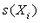is the standard devidation of the ith column of the dataset

Select “ED: mean centering divided by StdDev” in the same table: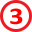Quantile Normalisation: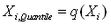Select “ED:quantile [0..1]” in the same table: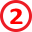q(x) is an operator that gives as output a number between 0 and 1.

The q(x) operator on a column C of the database is computed this way:

Sort in increasing order all the numbers in C and remove all the duplicates. We obtain a new “sorted” column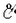.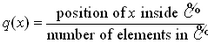Thus, q(x) is zero when x is the smallest number inside the column C

and q(x) is one  when x is the largest number inside the column C.

The Euclidean-distance between two rows of the dataset (between the two points A and B) is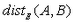and is defined this way: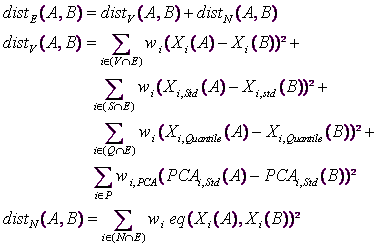... where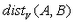is a distance based on variables that only contains continuous numbers (values)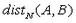is a distance based on nominal variables only.

E is the set of “active” variables to include inside the Euclidean distance-definition.

V is the set of non-normalized continuous variables

S is the set of continuous variables that have been normalized using the “Standard” normalization

Q is the set of continuous variables that have been normalized using the “Quantile” normalization

N is the set of Nominal variables

P is the set of “active” PCA axises

eq(x,y) is an operator that returns one if the string ‘x’ equals ‘y’ and zero otherwise.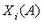is the content of the column ‘i’ and row ‘A’ of the datasetand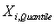have been defined on the previous page.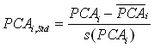is the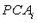axis after a “Standard” normalization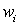and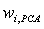are user-specified (column-)weights.

The default values are: the sets V,S,Q,E are empty, the set S contains all the continuous variables, the set P contains the first ten PCA axises, the weights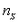and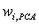are all one.

If some variables are included inside a “cosine-distance” (the C set is non-empty), then the distance-definition between two points A and B is: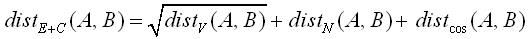...where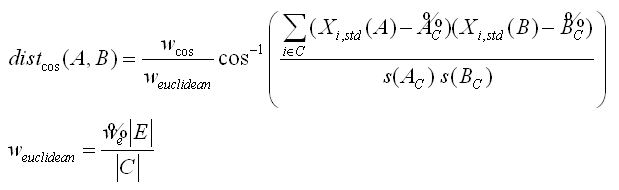... and where C is the set of variables that are, at the same time, included inside the “cosine-distance” (column “normalize”) and that are “active” (column “In K-Means”).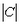is the number of variables inside the set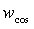is a user-defined parameter that specified the relative weight of the cosine-distance compared to the Euclidean-distance.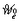is the average of the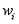anduser-specified weights included inside the Euclidean-distance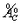is the mean of a vector composed by the value of the columns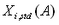with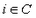on the row A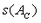is the Standard deviation of a vector composed by the value of the columnswithon the row A

The default values are: the set C is empty, the weight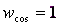You can define the sets E,C here:You can define the sets V,S,Q,C here:You can define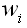here:You can define the sets E,C,P here:You can definehere: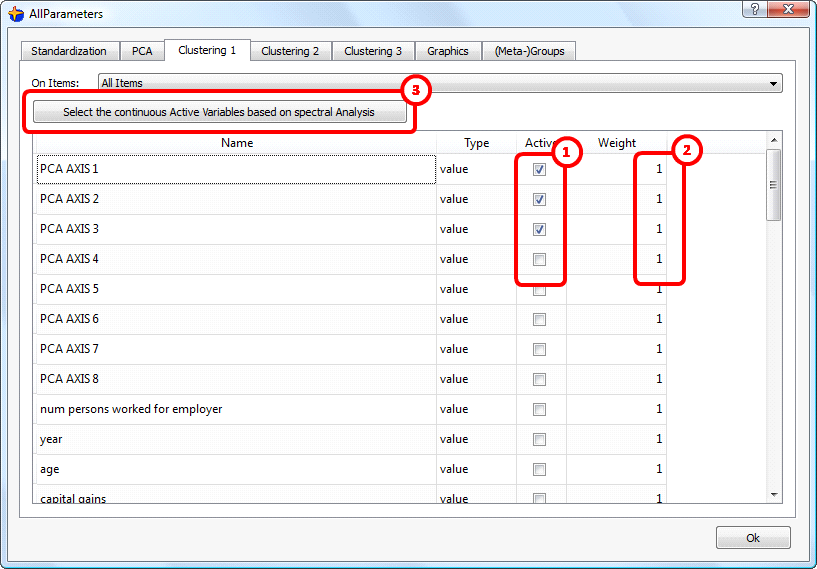To help you to select the set P of the active PCA axises, you can click here:When you click the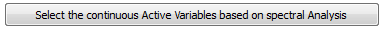button, the following window appears: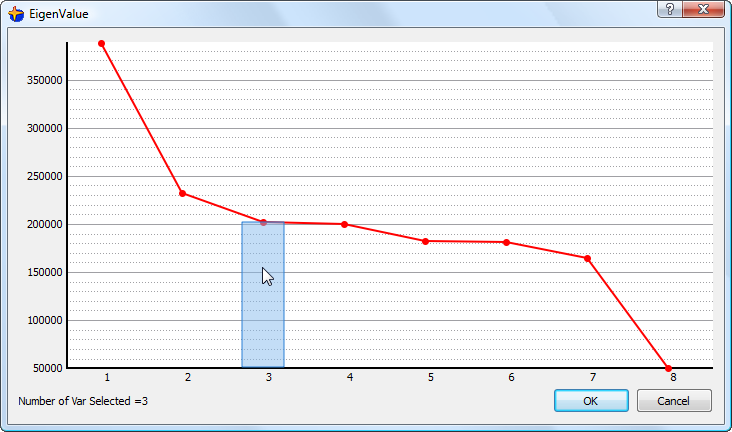Please refer to the section 2.2.5 to know how to select to right number of PCA axises to include inside your “distance-definition”.

The default, initial setting for the “distance-definition” is equivalent to the following “distance-definition”: